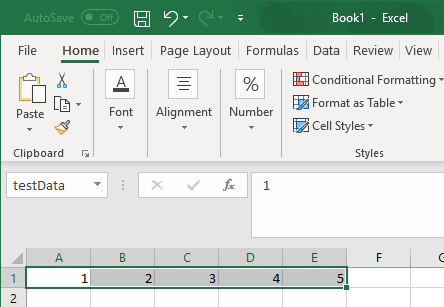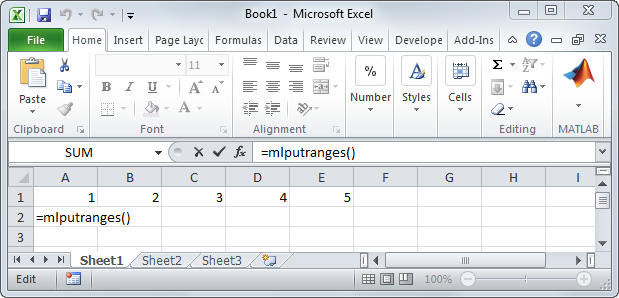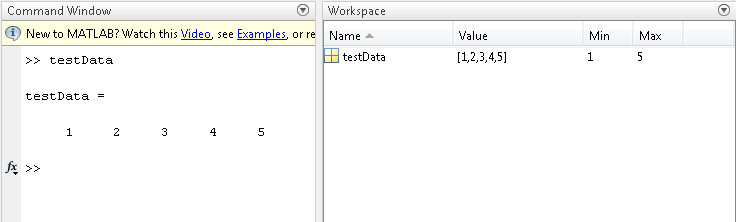Documentation

MLPutRanges

Send data in Microsoft Excel named ranges to MATLAB

Description

example

= MLPutRanges() writes the named cell ranges in a Microsoft® Excel® worksheet into MATLAB® variables. The variables are named with the same name specified by the cell range name in Microsoft Excel. To use this syntax, right-click in any Microsoft Excel cell, enter this syntax, and press Enter.

example

MLPutRanges writes the named cell ranges in a Microsoft Excel worksheet into MATLAB variables. The variables are named with the same name specified by the cell range name in Microsoft Excel. Use this syntax when working directly in a Microsoft Visual Basic® macro.

example

out = MLPutRanges() returns the named cell ranges in a Microsoft Excel worksheet into MATLAB variables. The variables are named with the same name specified by the cell range name in Microsoft Excel. In this case, out specifies whether the MLPutRanges function executed successfully. Use this syntax when working directly in a Microsoft Visual Basic macro.

Examples

collapse all

Define a name for a range of cells from cell A1 through cell E1. For instructions about defining names, see Excel Help and enter the search term: define and use names in formulas.

The name of the range of cells testData appears in the Name Box.To send data in the named range in the current worksheet to MATLAB, execute the function inside a worksheet cell.

= MLPutRanges()After pressing Enter, Microsoft Excel exports the named range testData to the MATLAB variable testData in the MATLAB workspace.Call the function to send data in the named ranges in the current worksheet to MATLAB.

MLPutRanges

Call the function to send data in the named ranges in the current worksheet to MATLAB.

out = MLPutRanges()

out returns 0 if the function succeeded or a string with the corresponding error code if the function failed.

Output Arguments

collapse all

Status for execution of MLPutRanges, returned as 0 if the function succeeded, or a string containing an error code.

Introduced in R2013b